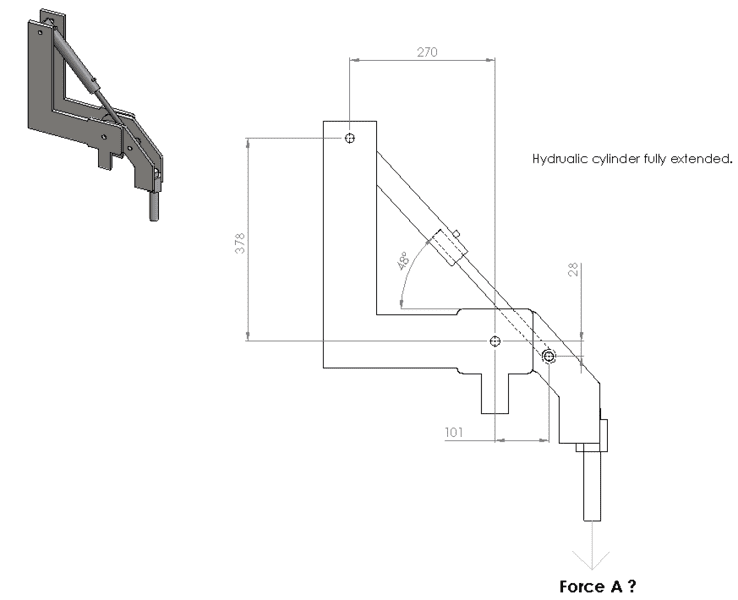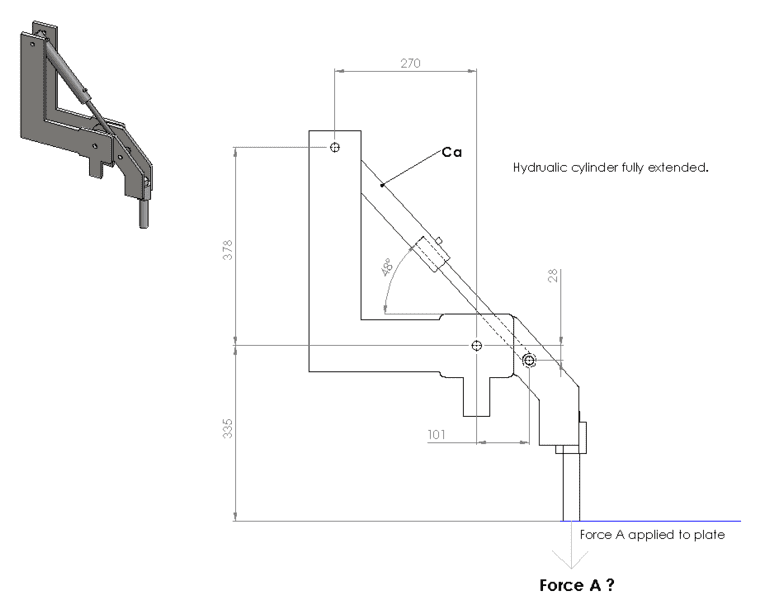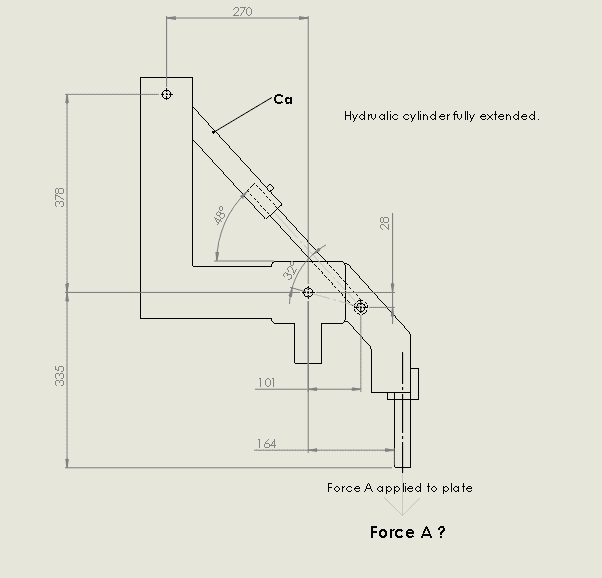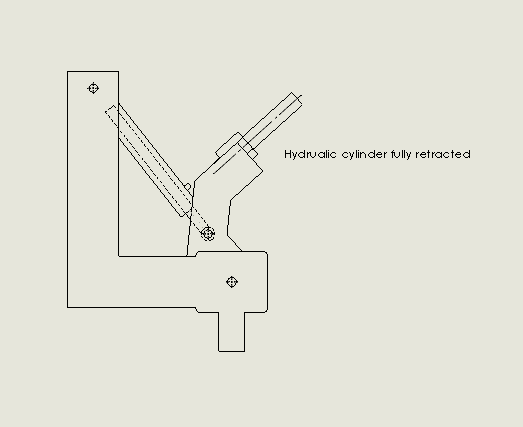# Force Calculation -- Hydraulic cylinder and a rotating arm

• IanPibworth
The resulting force will vary depending upon the cylinder angle at the point of contact of your pressing pin on your material.For the 48° position shown, the vertical component of force the cylinder applies to the lower arm equals the cylinder force x sin 48°; the resulting torque on the arm then equals that vertical force x 101 (the horizontal distance from the arm pivot pin c/c to the cylinder connecting pin c/l); the resulting vertical force applied to the vertical pressing pin then equals that torque divided by the horizontal distance from the arm pivot c/l to the vertical pressing pin c/l (which is missing from your figure).The resulting force will vary depending upon the cylinder angle at the point of contact of your pressing

#### IanPibworth

Hi There,

Long time reader, first time poster..

I hope someone out there can help me. I'm designing an add-on to an existing piece of equipment and I'm unsure of how to calculate the resultant force output from a hydraulic cylinder.

On a straight push, the chosen hydraulic cylinder has an extension force of 16.8KN.

BUT, what will be the output force (A) in the configuration sketched below (Dimensions in mm). I know for an angled push I can calculate the X or Y component of the force by multiplying by Sin or Cos of the angle accordingly but I don't think its that simple in this instance.

Its been many years since I've done this sort of thing so I'm getting nowhere, any and all help welcome.

Cheers,
Ian#### Attachments

The sum of the torques about the axis of rotation = 0. There are several (slightly different) tactical approaches. I would express both forces (cylinder, A) in radial and tangent components (so I could ignore the radial). You're missing some dimensions (location where force A is applied), no matter what method you choose.

There is only 1 cylinder Ca. Force A is applied by the cylinder rotating the arm down. An adjustable length screw contacts a flat surface.

Missing Dimension added below. This dimension will change depending on the thickness of the plate the force is being applied to (range of about 30mm)#### Attachments

•CWatters
Understood. You still need a dim for the 'x' location of force 'A' (you added only the 'Y' location).

Welcome to the PF, @IanPibworthCan you say a bit more about what this mechanism is doing? The hydraulic cylinder would seem to have very poor leverage if your intention is to rotate the arm about its pivot point...

The resulting force will vary depending upon the cylinder angle at the point of contact of your pressing pin on your material.
For the 48° position shown, the vertical component of force the cylinder applies to the lower arm equals the cylinder force x sin 48°; the resulting torque on the arm then equals that vertical force x 101 (the horizontal distance from the arm pivot pin c/c to the cylinder connecting pin c/l); the resulting vertical force applied to the vertical pressing pin then equals that torque divided by the horizontal distance from the arm pivot c/l to the vertical pressing pin c/l (which is missing from your figure).

•IanPibworth

My intention is to have a series of these arms to hold a pipe in position as an automatic welding machine goes past. Each arm would move out of the way in turn to allow welding. At the moment I have a manual machine, each arm is held in position by a locking pin and the extending screw is tightened onto the pipe to prevent movement during welding. The idea is to automate this process. For info, the pipe is also supported separately, the moveable legs only aid line-up at the faces to be welded.

I need the pivot point in such a place so that the arm is completely out of the way when retracted. See second picture.#### Attachments

JBA said:
The resulting force will vary depending upon the cylinder angle at the point of contact of your pressing pin on your material.
For the 48° position shown, the vertical component of force the cylinder applies to the lower arm equals the cylinder force x sin 48°; the resulting torque on the arm then equals that vertical force x 101 (the horizontal distance from the arm pivot pin c/c to the cylinder connecting pin c/l); the resulting vertical force applied to the vertical pressing pin then equals that torque divided by the horizontal distance from the arm pivot c/l to the vertical pressing pin c/l (which is missing from your figure).

Thanks for the reply, would you then agree with the following calculation?

Sin48 x 16.8e3 = 12.48e3 N

12.48e3x101e-3 = 1260.9 N/m

If the horizontal distance between the arm pivot and the press pin is 180mm then:

1260.9/180e-3 = 7005N (715Kg)

That appears to be correct.

One observation regarding the retracted position alignment of the cylinder rod pin with the direct line between the cylinder mounting pin and the pivot pin. In that position, there is an almost negligible of the cylinder force that is directed toward starting the rotation of the arm and a large amount of direct force from the cylinder directly on the pivot pin which could result in wearing of the pin joint. Additionally, this can result in a very rapid acceleration of the pivot arm through its closing stroke unless the hydraulic feed rate to the cylinder is controlled.
I would recommend that you consider moving the cylinder rod pin a bit further to the right to increase a bit its offset from the direct line between the cylinder mounting pin and the pivot pin; and, carefully control your cylinder inlet flow rate to prevent an almost snap action closing of your clamp.

One note about your calculation units, the torque should be stated as N-m, not N/m

•jrmichler
I think that the force is more like 5250 N (535 Kg). The horizontal component of the cylinder has to be accounted for, as it is not directed into the point of rotation.

•IanPibworth
The horizontal component of the cylinder force acts as a horizontal force to the right on the arm pivot pin and the bottom end of the clamping pin. The total horizontal component of the cylinder force is Cos 48 x 16.8e3 = 11.2e3 N and that horizontal reaction is divided between the pivot pin and the bottom of the pressing pin by their respective lever ratios based upon the vertical distances of those two points from the rod connecting pin. The resulting horizontal force on the arm pivot is ((355-28)/355) x 11.2e3 = 10.32e3 and the horizontal reaction at the bottom of the loading pin is 28/355 x 11.2e3 = 0.88e3 the sum of the two reaction forces equals 10.32e3 + 0.88e3 = 11.2e3 as would be expected.

That same lever ratio process with the vertical component of the cylinder load can also be used to determine the vertical reaction load on the arm pivot pin.

Edit: If the horizontal reaction at the bottom of the pin concerns you (it would tend to push the pipe sideways if it no restrained by a side plate guide; then you could move the cylinder rod pin upward to a directly horizontal position from the lever pivot when the clamp is closed (with a small loss of clamping force) to eliminate the horizontal force at the bottom of the pressing pin.

I stand by my answer. I don't believe that your technique respects the constraint imposed by the pivot pin.

I prefer a slightly different way to do these type of calculations.
1) Draw a centerline down the center of the cylinder.
2) Measure the perpendicular distance from that centerline to the center of the pivot. Most, if not all, CAD programs have ways to make this measurement. Or you can measure it with a ruler.
3) Cylinder force times perpendicular distance equals moment applied to the arm.
4) Divide by the horizontal distance from the pivot to the center of the point of contact (164 mm plus half diameter of pin) to get force on the plate. Or, stated more correctly, sum of moments equals zero. The force against the plate is assumed to be vertical.
5) Pay close attention to what @JBA said in post #10. Add some friction to the pivot pin, and the whole mechanism could bind up when retracted.
6) Now that you have the forces, the force on the pivot pin is the vector sum of those forces.

•IanPibworth and CWatters
Dullard, It is ultimately your responsibility to select the method you believe is correct so I have no problem with that.

jrmichler, if you might find a bit of an available time, it would be helpful for you run the calculation with your method for comparison.

IanPibworth said:
On a straight push, the chosen hydraulic cylinder has an extension force of 16.8KN.
It appears to me that gravity is working to extend the cylinder. The cylinder will therefore need to apply a force in retraction which will be reduced from the available extension force by the sectional area of the rod.

I do not see how this can be solved without knowing the total mass and the centre of mass of the moving arm with load attached.
Apart from that, it appears to be a simple linkage problem.

Dullard, after carefully reviewing my prior analysis I am firmly convinced of its accuracy. As a result, I strongly recommend that you seek additional assistance in confirming your analysis. My main concern is that the your 535 kg value is 75% of my 715 kg result; and, that value will be basis for all stress analysis of your assembly's components, pin shear, frame arm bending, assembly mounting, etc.; and, if your analysis is incorrect, that can result in insufficient design strength of those components.

JBA-
I'm like you - just an interested bystander (not the builder).

Your method addresses the moment due to the vertical component of the cylinder force (correctly, IMO). That's a CW torque. What it does not do is account for the moment due to the horizontal component. That is applied below center, and as a result will produce a CCW torque - reducing the total torque, and the force 'A.'

•IanPibworth
Thanks for alerting me to my oversight. I realized that there was that counter torque during my analysis; but, greatly underestimated the magnitude of its effect on the A force, so I did not include it in that analysis; nor, in my recent review of that analysis.

Now that I have calculated that counter torque effect I find that my resulting A force result is 5364N. So Dullard's 5250N is clearly the more correct; and, I am the one that has to go sit in the corner and wear the dunce cap for my lack of diligence by not including it in my original analysis.

That can be the cost when one makes "preconceived assumptions" about what is and what is not significant to address in an analysis.

I apologize to all, especially Dullard for my arrogant response to him in suggesting he "seek additional assistance" with his analysis.

•IanPibworth
No worries - you weren't arrogant (by my standards, anyway). This is a discussion forum - discussion is expected!

JBA said:
One observation regarding the retracted position alignment of the cylinder rod pin with the direct line between the cylinder mounting pin and the pivot pin. In that position, there is an almost negligible of the cylinder force that is directed toward starting the rotation of the arm and a large amount of direct force from the cylinder directly on the pivot pin which could result in wearing of the pin joint. Additionally, this can result in a very rapid acceleration of the pivot arm through its closing stroke unless the hydraulic feed rate to the cylinder is controlled.
I would recommend that you consider moving the cylinder rod pin a bit further to the right to increase a bit its offset from the direct line between the cylinder mounting pin and the pivot pin; and, carefully control your cylinder inlet flow rate to prevent an almost snap action closing of your clamp.

One note about your calculation units, the torque should be stated as N-m, not N/m
JBA,

Thanks very much for your thoughts, this has all been very helpful.

Dullard said:
I think that the force is more like 5250 N (535 Kg). The horizontal component of the cylinder has to be accounted for, as it is not directed into the point of rotation.

Thank-you Dullard for hashing this out with JBA, clearly you both understand the problem and JBA just needed that nudge in the right direction.

Could you possibly outline the missing calculation I will need to complete this analysis with slightly different geometry?

jrmichler said:
I prefer a slightly different way to do these type of calculations.
1) Draw a centerline down the center of the cylinder.
2) Measure the perpendicular distance from that centerline to the center of the pivot. Most, if not all, CAD programs have ways to make this measurement. Or you can measure it with a ruler.
3) Cylinder force times perpendicular distance equals moment applied to the arm.
4) Divide by the horizontal distance from the pivot to the center of the point of contact (164 mm plus half diameter of pin) to get force on the plate. Or, stated more correctly, sum of moments equals zero. The force against the plate is assumed to be vertical.
5) Pay close attention to what @JBA said in post #10. Add some friction to the pivot pin, and the whole mechanism could bind up when retracted.
6) Now that you have the forces, the force on the pivot pin is the vector sum of those forces.

Using this method I get a similar answer to that explained by JBA and Dullard.

16.8e3 x 55e-3 (Point 2) = 924 N-m

924/180e-3 = 5133.3N (523kg)

Ian-

The calc that you did is correct, but incomplete. You calculated the torque due the vertical component of the cylinder force (clockwise about the pivot). You just need to repeat that calculation for the horizontal component of the cylinder force (cos 48, 28 mm). The force in your original calc is reduced by this quantity because the horiz component creates a CCW torque.

•IanPibworth